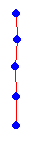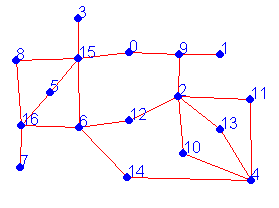Erich Prisner

# Wolf, goat, cabbage, ... II

### Variants: Larger boat

You need a larger boat to create more interesting problems. Try this larger boat version . You can also play it with my applet below.

Difficulty level 2:
1. Click at "A", "E", "I", "M", "Q" in the red box to the right.
2. Click on "Start".
Again you have to cross the river 7 times to get everybody to the right. But this time you can get two students in the boat at the same time.
Unfortunately your browser does not support Java applets.

### The Game GraphLook at the students in the red box at the beginning of the setup phase. A and B would fight. B and C would fight. A and E would fight. The complete list of all students in the red box, together with the complete list of all hostilities is called the red box graph. The students are the vertices, and each hostility, involving two students, forms an edge. Graphs are usually visualized by representing the vertices by dots in the plane, and connecting adjacent dots by a (not necessarily straight) line. See a picture of the red box graph on the right. A graph is a set of so-called vertices, and a set of unordered pairs of vertices, the so-called edges.Now we choose several students by clicking on them in the setup phase. Some hostilities will never play a role in our particular game, namely those where one of its two participants is not chosen. On the other hand, if we choose two enemies, we have the hostility. So by choosing our students, the vertices of our graph, we automatically choose the edges of our graph. The chosen students/vertices and the generated hostilities/edges form our game graph. In graph-theoretical terminology, the game graph is an induced subgraph of the red box graph. For our example above, the game graph looks as follows: The induced subgraph, induced by a subset W of the vertces, contains all edges that have both ends in W.
 The grid-like red-box graph above is a special bipartite graph. The coloring of the red-box graph above would be the well-known checkerboard pattern. The vertices of a bipartite graph can be colored with two colors such that no vertices of the same color are adjacent.

### Possible Beginnings

 Assume we have a k person boat. For which game graphs can we make at least the first move? There must be at most k students (vertices) such that, after moving them to the right the left bank becomes peaceful. In other words, removing the vertices in the game graph would induce an edgeless graph. Therefore we can make our first move exactly if the game graph has some vertex-edge covering of at most k vertices. A vertex-edge covering of a graph is a subset of the vertex set such that deleting these vertex induces an edgeless graph-

What about really large boats? Are you interested to find out how to decide the question whether a first move is possible for large k?

Question 2: What about the game graph shown on the right? How large a boat do we need?

 It turns out that this question is in general a really difficult one, but for bipartite game graphs, like the one shown, it is related to several other parameters and can be computed rather quickly (at least in polynomial time). Take a digression into the important topic of matchings in bipartite graphs.Research Question: Now we know when we may make a first move. Is it also possible to describe those game graphs where the puzzle can be solved?

previous page next page

Erich Prisner, 2002-2004